# Vertex maps on graphs-trace theorems

## Abstract

The paper proves two theorems concerning the traces of Oriented Markov Matrices of vertex maps on graphs. These are then used to give a Sharkoksky-type result for maps that are homotopic to the identity and that flip at least one edge.

2000 Mathematics Subject Classification

37E15, 37E25

## 1. Introduction

A vertex map on a graph is a continuous map that permutes the vertices. Given a vertex map, the periods of the periodic orbits can be computed; giving a subset of the positive integers. One of the basic questions of combinatorial dynamics for vertex maps is to determine which subsets of the positive integers can be obtained in this way. Sharkovsky's theorem  is a well-known result that answers the question when the underlying graph is topologically an interval and the vertices all belong to the same periodic orbit. In [2, 3] a Sharkovsky-type theorem was proved for trees.

In the vertex map papers, a standard method is to construct a matrix, called the Oriented Markov Matrix. The entries along main diagonal of the matrix give information about periodic orbits. In particular, the diagonal entries of the matrix raised to the n th power give information about the periodic orbits with period n. Thus the trace of powers of the matrix becomes important.

In this paper, two results concerning the trace of powers of the Oriented Markov Matrix are proved. The first shows that the trace is a homotopical invariant. The second shows how the trace can be calculated from the number of edges in the graph and the number of vertices that are not fixed by the vertex map. These results follow from Hopf's proof of the Lefschetz Fixed Point Theorem. However, since graphs are homologically very simple, it is possible to give elementary proofs, which we do.

The trace theorems are then used to prove the following theorem.

Theorem 1. Let G be a graph with v vertices. Let f be a vertex map on G that is homotopic to the identity and such that the vertices form one periodic orbit. Suppose f flips an edge.

1. (1)

If v is not a divisor of 2 k then f has a periodic point with period 2 k .

2. (2)

If v = 2 pq, where q > 1 is odd and p ≥ 0, then f has a periodic point with period 2 pr for any rq.

(Throughout the paper we will say that the period of a periodic point is the number of distinct points in its orbit. This is often referred to as the minimal or least period.)

It should be noted that all maps on trees are homotopic to the identity. Indeed, the proof of this theorem should be compared to the proofs of Sharkovsky Theorem in  and the theorem on trees in .

In this paper we study maps for which the vertices are permuted by the map. Maps on trees and graphs can be studied without this restriction. For general maps on trees see . For general maps on graphs see [6, 7].

## 2. Graphs

An edge is a space homeomorphic to the closed interval [0, 1]. The boundary points of the edges are vertices. An edge is not allowed to have a vertex as an interior point. The intersection of two distinct edges is empty, consists of one vertex or of two vertices. We assume that we have a finite number e of edges and v of vertices. The graph is the union of vertices and edges. We assume that graphs are connected and closed. We allow the possibility that there is more than one edge between the same two vertices. We also allow the possibility that an edge connects a vertex to itself (in this case the edge is homeomorphic to a circle).

We will label the vertices V1 to V v ; in diagrams we will just label them with the integers 1 to v. If V k and V l are vertices and there is an edge between them we will choose an orientation or direction on the edge and consider it as a directed edge from one vertex to the other. We will label the positively oriented edges as E1, ..., E e . If E p is an edge from V k to V l then the same edge, but with the opposite (negative) orientation that goes from V l to V k will be denoted by -E p . We will call V k the first vertex of E p and Vl the second vertex. (So the first vertex of E p equals the second vertex of -E p and vice versa.)

Given any two vertices, V r and V s , a path from Vr to V s is a sequence of edges E1 ... E q where the first vertex of E1 is V r , the second vertex of E q is V s and the second vertex of El equals the first vertex of El+1for 1 ≤ lq - 1. If E p and -E p are two consecutive edges in a path we can obtain a shorter path by omitting these two edges. We will call this a contraction of the path. Given any path from vertex V r to vertex V s we can form a sequence of contractions resulting in a unique path that cannot be contracted further. We call this resulting path fully contracted. Given a path P in the graph we will let FC(P) denote the fully contracted path that is obtained from P.

We define a circle in graph G to be a closed path that has no repeated vertices. Thus in Figure 1, E1E2 - E3 and E3E4E5E6 are circles.

## 3. Linearization of vertex maps

A vertex map of a graph G is a continuous map from G to itself with the property that the vertices of G are permuted by f. In this section we will define the linearization of a vertex map.

Suppose θ is a permutation on 1, ..., v and that f is a map from graph G to itself with the property that f(V i ) = Vθ(i)for 1 ≤ iv. Then we define L f , the linearization of f, to be the continuous map from G to itself given by L f maps the edge E with first vertex V k and second vertex V l linearly onto the fully contracted path from Vθ(k)to Vθ (l)that is homotopic to f(E), where f(E) is considered a path parameterized by E and the homotopy fixes both Vθ (k)and Vθ(l). This is done for each edge E in the graph. We make the observation that it is possible for a fully contracted path to contain positive or negative multiples of circles, but the loop based at Vθ(k)obtained by going to Vθ(l)along the path given by L f (parameterized by E) and then back to Vθ(k)by the path given by f (parameterized by -E) will be contractable to a point.

For maps of the interval or of trees this linearized map is often called the connect-the-dot map associated to θ. For trees (and intervals) f is always homotopic to the identity and so there is a unique linearization associated to θ. However, for graphs in general, the linearization depends on both θ and the homotopy type of the map f on the edges.

Example 1. Figure 1 shows a graph G. In this case the map f: GG permutes the vertices by f(V i ) = Vθ(i)where θ S n is given by θ = (13)(245), and f(E1) = E3E1E2E4, f(E2) = E5, f(E3) = E4E5, f(E4) = E6E1, f(E5) = E1 and f(E6) = E3.

It is straightforward to check thatand## 4. Oriented Markov graphs

We now define the Oriented Markov Graph associated to the graph G and L f . This is a directed graph on e vertices. Each of the directed edges also has either a positive or negative sign attached. It is defined as follows: the vertices of the Markov graph correspond to the edges of the graph (we will abuse notation and also denote these vertices by E1, ..., E e , the context should make it clear whether E k refers to the edge in the original graph G or the corresponding vertex in the Markov graph); we draw a positive directed edge from vertex E i to vertex E j for each occurrence of E j in L f (E i ); we draw a negative directed edge from vertex E i to vertex E j for each occurrence of -E j in L f (E i ). We will denote this Oriented Markov Graph by OMG(f).

Sometimes we will not need to use the fact that the directed edges in OMG(f) have a positive or negative sign attached. We will use the notation E k E j to indicate that there is a directed edge from E k to E j .

Letbe a path in the Oriented Markov Graph. This path will be have positive orientation if the number of negative directed edges is even and negative orientation if the number of negative directed edges is odd.

Lemma 1. Let f be a vertex map on G. Suppose thatis a path in OMG(f). Then. If the pathin OMG(f) has positive orientation thenappears in the path (in G). If the pathin OMG(f) has negative orientation thenappears in the path (in G).

Conversely, ifappears in the path (in G)then there is a positively oriented path (in OMG(f)) of length m fromto; and ifappears in the path (in G)then there is a positively oriented path (in OMG(f)) of length m fromto.

The following results are standard (see  or , for example, for a formal proof).

Lemma 2. Let f be a vertex map on G. Suppose that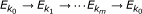is a loop in OMG(f). Then there exists a periodic point x of L f withsuch thatfor r = 0, 1, ..., m. Conversely, if x is a periodic point in L f of period m + 1 and if x is not a vertex then there exists a loopin OMG(f) such thatfor r = 0, 1, ..., m.

As in  we can change L f to f in the first half of the above lemma to obtain the following.

Lemma 3. Let f be a vertex map on G. Suppose thatis a loop in OMG(f). Then there exists a periodic point x of f with fm+1(x) = x such thatfor r = 0, 1, ..., m.

If the loopin the above statement is not a repetition of a shorter loop then we will say that it is a non-repetitive loop of length m + 1.

The importance of non-repetitive loops in the Oriented Markov Graph is the following extension of Lemma 2.

Lemma 4. Let f be a vertex map on G. Suppose thatis a non-repetitive loop in OMG(f). Then there exists a periodic point x of f with fm+1(x) = x such thatfor r = 0, 1, ..., m. If x is not a vertex of G, then x has minimum period of m + 1.

Note that if the non-repetitive loop in the statement above has negative orientation, then there must be a closed subinterval ofthat gets mapped with negative orientation ontounder fm+1. This means that the periodic point x can be chosen to lie in the interiorand so it definitely is not a vertex of G.

## 5. Oriented Markov matrices

Suppose that f is a vertex map on G. Then we define the Oriented Markov Matrix of f, denoted M(f), to be the e × e matrix with M(f) i,j equal to the number of positive directed edges from E j to E i minus the number of the negative edges from E j to E i . This is equivalent to saying that M(f)i,jis equal to the number of times that E i appears in the path L f (E j ) minus the number of times that -E i appears in the path L f (E j ).

Induction gives:

Theorem 2. Let f be a vertex map on G. Then ((M(f)) n ) i,j is the number of positively oriented paths (in OMG(f)) from E j to E i minus the sum of negatively oriented paths (in OMG(f)) from E j to E i .

Observe that to obtainfromwe contract pathsto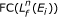and that each time a contraction is formed we lose the same edge with both positive and negative orientation, so for each j the number of times E j appears minus the number of times that -E j appears is the same in bothand. So.

Theorem 3. Let f be a vertex map on G. Thenfor any positive integer n.

Example 1 continued. In Example 1 the oriented Markov matrix is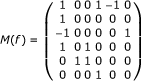andThe first columns of these matrices give information about L f (E1) and, respectively.

## 6. Homotopic maps and the trace

In this paper we have used language that is typically used in one-dimensional dynamics. However, many of these ideas also belong to algebraic topology and can be expressed in that language. In particular, the underlying space is a one-dimensional simplicial complex; the map L f is essentially a simplicial transformation; and M(f) is the matrix representation of the induced map on one-chains.

The goal of the next two sections is to prove results concerning the trace of M(f). These results follow simply from Hopf's trace formula which states that the alternating sum of traces in homology is equal to the alternating sum of traces on the chains. However, homological arguments are simple in the one-dimensional case, and we give short elementary proofs. (The change of basis in Section 7:2 comes from Hopf's proof of the Lefschetz Fixed Point Theorem given in .)

Theorem 4. Let f be a vertex map on G. If f is homotopic to g then Trace(M(f)) = Trace(M(g)).

Proof. Suppose that E r = [V k , V l ] is an edge in G. Then for any linearized map L with L(V i ) = f(V i ) for 1 ≤ iv we know that L will map [V k , V l ] to a fully contracted path from f(V k ) to f(V l ). In general there are infinitely many possible fully contracted paths from f(V k ) to f(Vl). We observe what happens to the Mr,rentry in the Markov matrix when we change from one fully contracted path from f(V k ) to f(V l ) to another. Notice that the Mr,rentry only changes if we change the number of times that the path goes around a circle that contains E r . Now, the number of times that a circle is wrapped around itself is a homotopy invariant. Thus if we change the fully contracted path from f(V k ) to V f (V l ) by adding n copies of a circle containing E r , thus changing the Mr,rentry by n and we want to end up with a map that is homotopic to the original one, then we have to make changes to the fully contracted paths that are the images of the other edges in the circle so that they now, in total, add -n copies of the circle. The only way that this can be done is by changing the image of other edges in the circle by adding complete copies of the circle. Notice that though the diagonal entries in the Markov matrix corresponding to a circle may change the sum of these entries must be constant if we wish to preserve the homotopy type of the map. This means that the trace of M(f) equals the trace of M(g) whenever f and g are homotopic.    □

## 7. Maps homotopic to the identity--the trace theorem

Suppose that f : GG is a map with the property that f fixes exactly r vertices, i.e., there are exactly r vertices with the property that f(V) = V. This means that there are exactly v - r vertices that are unfixed. We will let U(f, G) denote the number of unfixed vertices of G under f. In this section we show that if f : GG is homotopic to the identity then the trace of M(L f ) equals e - U(f, G).

Theorem (trace theorem). Let f be a vertex map on G. Suppose that f is homotopic to the identity map on G. Then Trace(M(f)) = e - U(f, G).

The proof is given below. The outline is to first prove it for trees, which is straightforward. Then we need to calculate the trace of M(f) for general graphs. This is done by introducing the chain group and seeing that the matrix induces a homomorphism. Then a new basis is chosen for the chain group. The induced map on chains gives a new matrix that is similar to M(f), but such that it is simple to calculate its trace.

### 7.1. Proof of the Trace theorem when G is a tree

First the case when G is a tree. (This is a slight generalization of a result in , where it was proved for the case when none of the vertices are fixed by f.)

Proof. For each unfixed vertex, V i there is a fully contracted path from V i to f(V i ) that will be denoted P(V i ). The initial vertex in this path is V i . At V i draw an arrow (direction arrow) pointing along the first edge of P(V i ). We repeat this process for each of the unfixed vertices in G. If a vertex V is fixed then no direction arrows are drawn at V.

Observe that an edge E i contains two direction arrows if and only if - E i is in L f (E i ). Also observe that E i contains no direction arrows if and only if E i is in L f (E i ). Finally, an edge contains one direction arrow if and only if L f (E i ) does not contain either E i or - E i . Notice that the number of arrows on the edge E i is exactly 1 - M(f)i,i. So the total number of arrows is. However, there are exactly U(f, G) arrows in G, one for each unfixed vertex, so U(f, G) = e - Trace(M(f)).

### 7.2. Chain groups

An integral 1-chain is a formal sum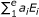, where a i are integers. Addition of chains is defined byThus 1-chains form a free abelian group with e generators. The coordinate vector of a 1-chainrelative to the ordered basis (E1, E2, ..., E e ) is [a1, a2, ..., a e ] T .

The Oriented Markov Matrix, M(f), defines a homomorphism from the chain group to itself by sending the 1-chain with coordinate vector v to M(f)v. Notice that this is entirely consistent with the previous interpretation of M(f), in that for any path P in G we can find the coordinate vector v; the coordinate vector of the image of P under L f is M(f)v.

### 7.3. Spanning trees-change of basis

Let T denote a spanning tree for G. This contains v - 1 edges, we will denote them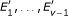. There are then e + 1 - v edges in G that do not appear in T. For each edge, E, that is in G, but not T choose a circle in G that consists of E plus edges in T. In this way e + 1 - v circles are obtained, denoted C1, ..., Ce+1-v.

Notice that if E i is the edge in C i that does not belong to T, then there exist paths P1 and P2 in T such that E i = FC(P1C i P2). This means that any fully contracted path in G can be expressed as the contraction of a path that is written only using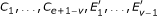.

We now consider the matrix, N, that represents the homomorphism on 1-chains with respect to the ordered basis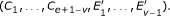Since f is homotopic to the identity and C i is a circle, we have L f (C i ) = C i . This means that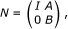where I is the (e + 1 - v) × (e + 1 - v) identity matrix and 0 is the (v - 1) × (e + v - 1) zero matrix.

The matrices M(f) and N are similar, because they represent the same homomorphism with respect to different bases. This means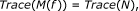but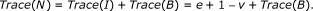To complete the proof we must calculate Trace(B).

### 7.4. Completion of the proof

For each edge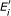in T we know that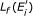can be written as a path using. Since circles both begin and end at the same vertex, notice that if we delete all the circles in this path we obtain a new path that lies entirely in T and has the same endpoints as L f (E i ). We define f' to be the map on T defined in this way. Note that f' is a vertex map on T with vertex permutation given by θ. Also note that M (f') = B. Thus by the proof for trees we know that Trace(B) = v - 1 - U (f, G).

In the previous section it was shown that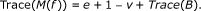So we obtain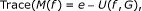which completes the proof.

### 7.5. Example 1 continued

In this subsection we illustrate the construction using Example 1.

We take as the spanning tree the tree formed by the edges E1, E3, E5 and E6. Then there are two circles. We let C1 = E3E4E5E6 and C2 = E1E2- E3. The new ordered basis for the chain group is (C1, C2, E1, E3, E5, E6). Notice that E2 = - E1C2E3 and E4 = -E3C1E-6-E5. So L f (E1) = -E3E1E2E4 = -E3E1 - E1C2E3 - E3C1 - E6- E5 which can be contracted to -E3C2C1- E6- E5. In this way we obtain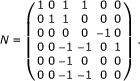The map f' on the spanning tree permutes the vertices in exactly the same way as f but maps the edges in the following way: f'(E1) = -E3 - E6 - E5, f'(E3) = -E3 - E6, f'(E5) = - E1 and f'(E6) = E3.

## 8. Vertices form one periodic orbit

In this section we study vertex maps where the vertices of G consist of one periodic orbit.

First, we state some basic results concerning the trace of the Oriented Markov Matrix. This is followed by some results for maps that are homotopic to the identity. Finally, we look at maps that are homotopic to the identity and that have at least one edge flipped, and show that we can obtain some results that are connected to the Sharkovsky ordering in this case.

Given a vertex map f, we will let θ denote the permutation on v elements defined by f (V i ) = Vθ(i)for i = 1, ..., v.

### 8.1. Basic trace results for maps that are homotopic to the identity

Suppose that the vertices of G consist of one periodic orbit. Then in this case, if k is not an integer multiple of v, then θk (i) ≠ i for i = 1, ... v; and that if k is an integer multiple of v then θk is the identity. This fact, along with the fact that f n is homotopic to the identity for any positive integer n, gives us the following corollary to the Trace theorem.

Corollary 5. Let G be a graph with e edges and v vertices. Suppose that f : GG is homotopic to the identity. Suppose that the vertices of G form one periodic orbit. Then:

1. (1)

Trace(M(f) k ) = e - v if k is not an integer multiple of v.

2. (2)

Trace(M(f) k ) = e if k is an integer multiple of v.

The following result follows immediately from Theorem 7 in .

Theorem 6. Let G be a graph with e edges and v vertices. Suppose that f : GG is homotopic to the identity. Suppose that the vertices of G form one periodic orbit. Then M(f) v = I, where I is the e × e identity matrix.

### 8.2. Edge flipping and the proof of Theorem 1

We say a vertex map f on a graph flips an edge E if - E appears in L f (E).

First we make the observation that if the underlying graph is a tree and that none of the vertices are fixed by the vertex map, then it must have this edge flipping property.

Lemma 5. If f is a vertex map on a tree that does not fix any vertices, then f flips an edge.

Proof. By Corollary 5, the trace of M (f) = -1. Thus M (f) must have an entry along the main diagonal that is a negative integer.    □

The following two lemmas plus Lemma 4 complete the proof of Theorem 1.

Lemma 6. Let G be a graph and f a vertex map from G to itself that is homotopic to the identity. Suppose that the vertices form one periodic orbit. Suppose f flips an edge. If v is not a divisor of 2 k, then OMG(f) has a non-repetitive loop of length 2 k with negative orientation.

Proof. Since f fl ips an edge, there must be at least one loop in the Markov graph that has length 1 and has negative orientation. Since Trace(M (f)) = e - v, there must be at least e - v + 1 loops of length 1 that have positive orientation. By going around each of these loops in the Markov graph twice we can see that there must be at least e - v + 2 loops of length 2 that have positive orientation. Since Trace(M (f)2) = e - v, there must be at least one loop of length 2 with negative orientation. Since it has negative orientation, it cannot be the repetition of a shorter loop. So the Markov graph of f has a non-repetitive loop of length 2 with negative orientation.

Now, for any positive integer k, consider loops of length 2 k in the Markov graph. If j < k, and the Markov graph has a non-repetitiveloop of length 2 j , then this gives a loop of length 2 k by going around it 2 k-j times. Since 2 k-j is even, this repetitive loop of length 2 k must have positive orientation. From the argument above, we know there are at least e - v + 2 loops of length 2. This means that there must be at least e - v + 2 loops of length 2 k with positive orientation. Since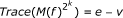, there must be a loop of length 2 k with negative orientation. This must be a non-repetitive loop because any repetitive loop of length 2 k consists of an even number of repetitions of the shorter loop and so must have positive orientation.    □

Lemma 7. Let G be a graph and f a map from G to itself that is homotopic to the identity. Suppose that the vertices form one periodic orbit. Suppose f flips an edge. If v = 2 pq, where q > 1 is odd and p ≥ 0, then M(f) has a non-repetitive loop of length 2 pr for any rq.

Proof. Let r - q = 2 st, where t is odd. The previous lemma tells us that there must be a non-repetitive loop of length 2p+swith negative orientation. Let E i denote an edge in this loop. Then Theorem 6 tells us that. So there must be a loop of length 2pq from E i to itself with positive orientation. Note that this loop cannot be a repetition of the loop of length 2p+sbecause this loop has negative orientation and repeating it an odd number of times gives a loop with negative orientation. A non-repetitive loop of length 2 pr can be obtained by first going t times around the loop of length 2p+sand then going around the loop of length 2 pq once. Since t is odd, the resulting non-repetitive loop has negative orientation.    □

In the first subsection we show that the edge flipping property is essential to the conclusion of Theorem 1. Then in the next subsection we compare the partial ordering given by Theorem 1 to the orderings given when the underlying graphs are restricted to trees.

### 9.1. Edge flipping

Given a graph G, we let the Euler Characteristic be the number of vertices minus the number of edges. Vertex maps of trees (and the interval) that do not fix any of the vertices have the edge flipping property. The graphs in these cases have Euler characteristic of 1. However, if the Euler characteristic is less than 1, then vertex maps need not flip edges and the set of periods of periodic points can consist of one or two numbers.

By considering rotations, it is clear that for maps of a circle, for any positive integer n it is possible to construct a map from the circle to itself that only has periodic points with period n and no other periods.

Lemma 8. Let G be a graph and f : GG a map that is homotopic to the identity and permutes the vertices. Then we can construct a graph G' by doubling one edge in G and a map f': G' → G' such that f' is homotopic to the identity and such that f' restricted to G equals f and such that f' has only one other periodic point-a fixed point.

Proof. Let E k be an edge in the graph G with vertices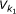and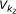Let P be a path in the graph fromto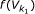. Then -E k followed by P followed by f(E k ), which we will denote by - E k Pf (E k ) gives a path fromto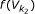. We form G' by adding a new edge, Ee+1that goes fromto. We define f' restricted to G to equal f and let f'(Ee+1) = PEe+1- E k Pf (E k ). We define f' to be linear on the subinterval of Ee+1that gets mapped onto Ee+1. Notice that this new map is homotopic to the identity and that the only new periodic point introduced is the fixed point in Ee+1.

The lemma above gives the second part of the next result. The first part follows from the Trace theorem which tells us that the trace of M(f) is non-zero and hence by Lemma 3, f must have a fixed point.

Theorem 7. Let G be a graph with v vertices. Suppose that f: GG is homotopic to the identity and has the property that the vertices form one periodic orbit. If ve, then f must have a fixed point.

Given any negative integer n we can construct a graph G with v vertices and e edges with v - e = n and a map f: GG homotopic to the identity with the property that the vertices form one periodic orbit and such that f has periodic orbits with period v and 1 and no other periods.

### 9.2. Orderings of the natural numbers

Theorem 1 gives a partial order on the natural numbers. This should be compared to the case when the underlying graph is assumed to be a tree in which case we get another partial order (see ); and to the case when the graph is topologically an interval in which case we get Sharkovsky's ordering, a total ordering. Note that in going from the Sharkovsky ordering to the tree ordering and then to the graph ordering, no relations are added, only relations are deleted. So the graph ordering is weaker than the tree ordering, and the tree ordering is weaker than Sharkovsky's ordering. Given a positive integer n, consider the set of natural numbers that are forced by n, {m |m n}, for each of the three orderings. If m n for the Sharkovsky ordering and m n for the graph ordering, then m < n. This means that for each n, the three sets {m |m n} only differ by a finite number of elements.

In both Sharkovsky's Theorem and the theorem on trees there is a result that is sometimes called the converse. This is the result that given any n in the ordering one can find an example of a vertex map that does not have a periodic point of period m for any m n. It is an open question as to whether the converse result holds for the graph ordering. We conjecture that it does, but this is little more than a guess.

Another natural question is to ask what happens in the case when the vertices of a vertex map form more than one periodic orbit. This is, of course, a harder question. For maps of the circle this leads to the study of rotation intervals. The beginnings of the generalization of this idea to graphs can be found in .

## References

1. Sharkovsky AN: Co-existence of the cycles of a continuous mapping of the line onto itself. Ukrain Math Zh 1964,16(1):61–71.

2. Bernhardt C: Vertex maps for trees: algebra and periods of periodic orbits. Disc Cts Dyn Sys 14(3):399–408.

3. Bernhardt C: A Sharkovsky theorem for vertex maps on trees. J Diff Equations Appl 2011,17(1):103–113. 10.1080/10236190902919327

4. Bernhardt C: A proof of Sharkovsky's theorem. J Differ Equations Appl 2003,9(3–4):373–379.

5. Alsedà L, Juher D, Mumbrú P: Periodic behavior on trees. Ergodic Theory Dyn Syst 2005,25(5):1373–1400. 10.1017/S0143385704000896

6. Leseduarte MC, Llibre J: On the set of periods for σ maps. Trans Am Math Soc 1995,347(12):4899–4942. 10.2307/2155068

7. Llibre J, Paraños J, Rodríguez JA: Periods for continuous self-maps of the figure-eight space. Dynamical systems and functional equations (Murcia, 2000). Int J Bifur Chaos Appl Sci Eng 2003,13(7):1743–1754. 10.1142/S0218127403007606

8. Block LS, Coppel WA: Dynamics in One Dimension. In Lecture Notes in Mathematical 1513. Springer, Berlin; 1992.

9. Alsedà L, Llibre J, Misiurewicz M: Combinatorial dynamics and Entropy in Dimension One. Advanced Series in Nonlinear Dynamics, World Scientific, Singapore 2000., 5:

10. Henle M: A Combinatorial Introduction to Topology. Dover Publications, Inc., New York; 1994:x+310.

11. Bernhardt C: Rotation matrices for vertex maps on graphs. J Differ Equations Appl 2011, in press.

## Acknowledgements

The author would like to thank the referee for many corrections and improvements to this paper.

## Author information

Authors

### Corresponding author

Correspondence to Chris Bernhardt.

### Competing interests

The author declares that they have no competing interests.

## Authors’ original submitted files for images

Below are the links to the authors’ original submitted files for images.

## Rights and permissions

Reprints and Permissions

Bernhardt, C. Vertex maps on graphs-trace theorems. Fixed Point Theory Appl 2011, 8 (2011). https://doi.org/10.1186/1687-1812-2011-8